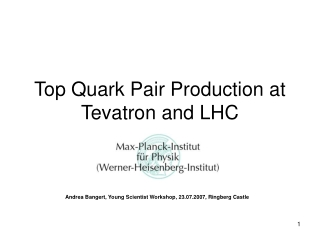DownloadDownload PresentationTop Quark Pair Production at Tevatron and LHC

# Top Quark Pair Production at Tevatron and LHC

Télécharger la présentation## Top Quark Pair Production at Tevatron and LHC

- - - - - - - - - - - - - - - - - - - - - - - - - - - E N D - - - - - - - - - - - - - - - - - - - - - - - - - - -
##### Presentation Transcript

1. Top Quark Pair Production at Tevatron and LHC Andrea Bangert, Young Scientist Workshop, 23.07.2007, Ringberg Castle

2. Overview • Top pair production • Pair production as test of perturbative QCD • Top decay • Cross section measurements at the Tevatron • Cross section measurements with the ATLAS detector • Conclusions

3. Top Production scale μ = μR = μF Parton Density Functions • Partonic cross section σij • Short-distance hard scattering. • Calculated to NLO in perturbative QCD. • Parton density functions f(x,μ2) • Non-perturbative but universal. • Determined from fits to experimental data. Measurement of σ serves as experimental test of pQCD.

4. Test of Perturbative QCD √s = 1.96 TeV

5. Top Decay • Top lifetime is τt~10-24 s • No top hadrons or bound states. • Γ(t→Wb) ~ 100% • Γ(W →lν)=1/3, Γ(W→qq’)=2/3 • Top events identified by decay products: • tt → Wb Wb → lvb lvb • “dileptonic” • Low background rates • Γ = 10.3% • tt → Wb Wb → lvb jjb • “lepton+jets” • Manageable background • Γ = 43.5% • tt → Wb Wb → jjb jjb • “hadronic” or “all jets” • High multijet background rates • Γ = 46.2%

6. Tevatron Measurements Kidonakis + Vogt: σ = 6.8 ± 0.6 pb Cacciari et al:σ = 6.7 ± 0.7 pb CDF,mt = 170 GeV: σ = 7.7 ± 0.9 pb CDF, mt = 175 GeV: σ = 7.3 ± 0.9 pb CDF Cross Section • Dilepton: Uncertainty on estimate of Z+jet, γ+jet backgrounds. • Lepton+jets: NN exploits kinematics and topology to distinguish ttbar from W+jet, QCD multijet backgrounds. • Lepton+jets: b-tagging using displaced secondary vertices. Uncertainty on εb-tag, W+Njet, QCD multijet backgrounds. • Lepton+jets: soft lepton b-tag. Uncertainty on εb-tag and mistag rate. • MET: Require missing ET. Selects tau+jets events. Trigger efficiency is dominant systematic uncertainty. • Hadronic: Uncertainty on QCD multijet rate, b-tag rate of multijet events.

7. Cross Section Measurement with ATLAS • LHC starts up in 2008. • L = 1033cm-2s-1 • ~1 top pair per second • Use ttbar analysis to understand the detector performance. • Extract jet energy scale. • Determine missing ET and b-tagging performance. The ATLAS Detector • NLO calculation: σ = 803 ± 90 pb • NLO + NLL: σ = 833 +52–39 pb • Bonciani, Catani, Mangano, Nason, hep-ph/9801375 A. Shibata

8. kT (D=0.4) Commissioning Analysis • Designed to perform first observation of top pair production with ATLAS. • L~100 pb-1 • 80000 top pairs. • Selection of semileptonic ttbar events: • one e or μ, 4 jets, missing ET. • Reconstruction: Take trijet combination with highest pT to represent t→Wb→jjb. • σ·Γ = 246.0 ± 3.5 (stat) pb • From Monte Carlo:σ·Γ = 248.5 pb

9. Top Quark and W Boson Masses • mt = 163.4 ± 1.6 (stat) GeV • Generated top mass is 175 GeV. • mW = 78.90 ± 0.5 GeV. • Generated W mass is 80.4 GeV. • Trijet combination with maximal pT represents t→Wb→jjb. • Dijet combination with maximal pT represents W→jj. • Fit mass distribution using Gaussian and polynomial; mean is fitted mass.

10. Summary • Measurement of σtt offers test of pQCD. • Theoretical calculation, √s = 1.96 TeV: σ = 6.7 ± 0.7 pb • CDF experiment: σ = 7.3 ± 0.9 pb • Theoretical calculation, √s = 14 TeV: σ = 833 +52–39 pb • ATLAS analyses currently performed using Monte Carlo generated events. • Optimization of event selection, evaluation of systematic errors is underway. • Measurement of σtt with ATLAS is scheduled for LHC startup in 2008.

11. Backup Slides

12. Tevatron Measurements L = 1032cm-2s-1, √s = 1.96 TeV

13. Atlantis Atlantis is an event display designed for the ATLAS experiment.

14. Comissioning Analysis Selection Cuts • MET > 20 GeV. • Exactly one e or μ with: • pT > 20 GeV • |η| < 2.5 • E(∆R<0.2)<6 GeV • In order to avoid the crack in the LAr calorimeter, exclude electrons with 1.35<|ηe|<1.57. • 3 jets with pT(j)>40 GeV. • 1 additional jet with pT(j4)>20 GeV. • |mjj - mW| < 10 GeV.

15. The Commissioning Analysis • Designed to perform first observation of top pair production with ATLAS. • L~100 pb-1 • 80000 top pairs. • Selection of semileptonic ttbar events: • one e or μ • 4 jets • missing ET. • Reconstruction: Take trijet combination with highest pT to represent t→Wb→jjb. • Discard event if no dijet combination W→jj has mjj~mW. Nikhef, Udine/ICTP, A.Shibata CSC sample #5200, event generator MC@NLO

16. Statistical Error on ε and σ • Error on efficiency: δε= √(ε (1- ε) / Ni) • δNe = √Ne, δNμ = √Nμ • δσe = δNe / Ldata εe • δσμ= δNμ/ Ldata εμ • δσ = √(δσe2 + δσμ2)